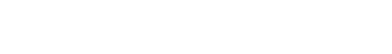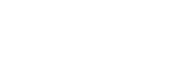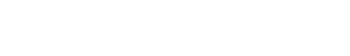# Physics Paper 2 Questions and Answers - Form 4 End Term 1 Exams 2021

PHYSICS
FORM 4
END TERM EXAMS
TERM 1 2021
PAPER 2
TIME 2 ½hrs

INSTRUCTIONS

• This paper consists of 2 sections: A and B
• Answer all the questions in these sections
• Mathematical tables and calculators may be used.

SECTION A (25 MARKS)
Answer ALL the questions in this section in the spaces provided.

1. Differentiate between nuclear fission and nuclear fusion as used in the study of physics. (2 marks)
2. The diagram below shows an electrical appliance connected to the mains.Name the colour for the leads A,B and C and state the purpose of the fuse. (3 marks)
3. The figure below shows a circuit diagram for a photocell.State the variation in the UV-light that will cause a decrease in the reading of the millimeter. (1 mark)
4. Diagram (a) below shows the position of the bright spot on the screen of a C.R.O when there is no signal on both Y and X- plate. Indicate on the diagram(b) when the Y-plate is connected to a.c signal and for (c) the X- plate is connected to a d.c signal i.e. the display on the screen. (3 marks)5. Using the band theory, differentiate between conductor and a semi-conductor . (1 mark)
6. The following is a decay series of Uranium 238.Determine the value of x and y. (2 marks)
7. A girl stands 2m in front of a plane mirror, the mirror is then moved 0.6m away from the girl. Determine the distance from the first position of the image to the second position of the image. (2 marks)
8. What is the ‘direction of the magnetic field’ at a point in the field? (1 mark)
9. State with a reason which type of reflector would be preferred for:
1. Underground parking area (1 mark)
2. Solar concentrators (1 mark)
10. Polarization and local action are the common defects in a simple cell. How are these defects minimized in the cell? (2 marks)
11. A man standing at the middle of two parallel walls fires a gun. He hears an echo after 1.5 seconds. Determine the distance of separation of the walls. (Velocity of sound is 340m/s) (2 marks)
12. A highly negatively charged rod is brought slowly towards the cap of a positively charged leaf electroscope. It is observed that the leaf initially collapses and then diverges. Explain the observations. (2 marks)
13. The figure below shows ray B1 incident through a glass block to air interface.B2 is the emergent ray of B1. Determine the refractive index of the glass block. (2 marks)

SECTION B (55 MARKS)

1.
1. What is meant by the term ‘photoelectric effect?’ (1 mark)
2. State any two factors that affect photoelectric effect. (1 mark)
3. The graph below shows stopping potential plotted against frequency, f.From the graph determine:
1. Planks constant. (3 marks)
2. Work function (2 marks)
4. A metal surface has a work function of 4.85eV. Calculate the maximum kinetic energy in joules of the electrons emitted when the metal is irradiated with uv light of frequency 1.75×1015Hz. (3 marks)
2.
1.
1. Explain why Carbon -14(614C)  is radioactive while Carbon – 12 (612C)  is not. (1 mark)
2. A radioactive isotope showed a count rate of 82 counts per second initially. After a time of 210 seconds, the count rate dropped to 19 counts per second. The average background countremained constant at 10 counts per second. What is the half-life of the material? (2 marks)
2. The figure below shows features of a diffusion cloud chamber used for detecting radiations from a radioactive source.Explain how the chamber works when a radioactive particle is introduced at the source. (2 marks)
3.
1. What is the purpose of solid carbon (IV) oxide. (1 mark)
2. State one advantage of the cloud chamber over a G.M tube as a detector of radioactive radiations. (1 mark)
4.
1. Using a diagram, explain how doping produces a p-type semi-conductor. (3 marks)
2. What is biasing? (1 mark)
3. The diagram below shows a circuit with a p-n junction and a very low power bulb.State with reason the observations made on the bulb when the switch is closed. (2 marks)
3. Some plane water waves were produced in a ripple tank. They pass from a region of deep water into a region of shallow water. The figure shows what the waves look like from above.1. State what happens at the boundary to:
1. The frequency of the waves. (1 mark)
2. The speed of the waves. (1 mark)
3. The wavelength of the waves. (1 mark)
2. The waves have a speed of 0.12ms-1 in the deep water wave crests are 0.08m apart in deep water. Calculate the frequency of the source producing the waves. (2 marks)
3. Arrange the following electromagnetic waves in order of their increasing wavelength.X-rays, gamma rays, ultra violet, visible light, infrared and microwaves. (2 marks)
4. Study the circuit below:1. Determine the combined resistance of the circuit. (2 marks)
2. Determine the current flowing in the circuit. (2 marks)
4.
1. The figure below shows a section a flexible wire carrying current perpendicularly out of the paper.The wire moves in the direction shown as current passes through it.
1. Label the polarities of the magnets A and B. (1 mark)
2. Explain the behaviour of the flexible wire. (2 marks)
2. The figure below shows an induction coil used to step-up voltage.1. State the difference between the induction coil and a step-up transformer. (1 mark)
2. Explain how voltage is stepped up by the induction coil. (3 marks)
3. The voltage is stepped up from 12V to 15kV. Determine the ratio of the secondary to primary coils in the induction coil. (1 mark)
4. The function of the capacitor is to eliminate sparking at the contacts. State why sparking occurs at the contacts. (1 mark)
5. State how the capacitor eliminates sparking. (1 mark)
5.
1. X-rays are used for detecting cracks inside metal beams.
1. State the type of the x-rays used. (1 mark)
2. Give a reason for your answer in (i) above. (1 mark)
2. Figure 4 shows the features of an x-rays tube.1. Name the parts labelled A and B. (1 mark)
2. Explain how a change in potential across PQ changes the intensity of the x-rays produced in the tube. (2 marks)
3. During the operation of the tube, the target becomes very hot. Explain how this heat is caused. (2 marks)
4. What property of lead makes it suitable for use as shielding material? (1 mark)
3. In a certain x-ray tube, the electrons are accelerated by a pd of 12000V. Assuming all the energy goes to produce x-rays, determine the frequency of the x-rays produced. (Planks constant h = 6.62 ×10-34 J and charge of an electron, e = 1.6 ×10-19 C) (3 marks)## MARKING SCHEME

SECTION A (25 MARKS)
Answer ALL the questions in this section in the spaces provided.

1. Differentiate between nuclear fission and nuclear fusion as used in the study of physics. (2 marks)
• Fission - Splitting of a large radioactive nuclide into almost two equal halves
• Fusion – Combining of into light nuclides to form one large fragment

2. The diagram below shows an electrical appliance connected to the mains.Name the colour for the leads A,B and C and state the purpose of the fuse. (3 marks)
1. - Brown red
2. - Yellow/ green
3. - Blue
3. The figure below shows a circuit diagram for a photocell.State the variation in the UV-light that will cause a decrease in the reading of the millimeter. (1 mark)
• Reduce intensity of light.

4. Diagram (a) below shows the position of the bright spot on the screen of a C.R.O when there is no signal on both Y and X- plate. Indicate on the diagram(b) when the Y-plate is connected to a.c signal and for (c) the X- plate is connected to a d.c signal i.e. the display on the screen. (3 marks)Ans5. Using the band theory, differentiate between conductor and a semi-conductor . (1 mark)
• For conductor – conduction band and valence band overlap.
• For semi- conductor – conduction band and valence band are separated by a narrow energy gap.

6. The following is a decay series of Uranium 238.Determine the value of x and y. (2 marks)
• X - 92
• Y - 234

7. A girl stands 2m in front of a plane mirror, the mirror is then moved 0.6m away from the girl. Determine the distance from the first position of the image to the second position of the image. (2 marks)
• 1.2cm

8. What is the ‘direction of the magnetic field’ at a point in the field? (1 mark)
• Direction in which a free North pole wound move in the field

9. State with a reason which type of reflector would be preferred for:
1. Underground parking area (1 mark)
• Convex

2. Solar concentrators (1 mark)
• Parabolic

10. Polarization and local action are the common defects in a simple cell. How are these defects minimized in the cell? (2 marks)
• Polarization – by adding Potassium dichromate
• Local action – coating the zinc plate with mercury

11. A man standing at the middle of two parallel walls fires a gun. He hears an echo after 1.5 seconds. Determine the distance of separation of the walls. (Velocity of sound is 340m/s) (2 marks)
• Distance = Velocity × time
= 340 × 1.5
= 510m

12. A highly negatively charged rod is brought slowly towards the cap of a positively charged leaf electroscope. It is observed that the leaf initially collapses and then diverges. Explain the observations. (2 marks)
• The rod repels negative charges to the leaf neutralizing the positive charge and the leaf collapse. As more negative charges are repelled down, the leaf acquires negative charge and rises

13. The figure below shows ray B1 incident through a glass block to air interface.B2 is the emergent ray of B1. Determine the refractive index of the glass block. (2 marks)
• ŋ = 1/sin⁡C
= 1/sin⁡41.8
= 1.499

SECTION B (55 MARKS)

1.
1. What is meant by the term ‘photoelectric effect?’ (1 mark)
• Photoelectric effect is the emission of photoelectrons from a metal surface when irradiated by a radiation of appropriate frequency.
2. State any two factors that affect photoelectric effect. (1 mark)
• Intensity
• Type of the metal

3. The graph below shows stopping potential plotted against frequency, f.From the graph determine:
1. Planks constant. (3 marks)
• Slope = h/e = (1.5 - 0)/(8.5-4.5 ×1014 )
h/(1.6 ×10-19 ) = 1.5/(4 ×1014 )
h = 6.0×10-34Js

2. Work function (2 marks)
• w = hfo
= 6.0×10-34×4.0×1014
= 2.4 ×10-19J

4. A metal surface has a work function of 4.85eV. Calculate the maximum kinetic energy in joules of the electrons emitted when the metal is irradiated with uv light of frequency 1.75×1015Hz. (3 marks)
• E = hf
hf = θ+K.E max.
6.0×10-34×1.75×1015
= 2.4×10-19+K.E max
K.E max = (6.0×10-34×1.75×1015 )- 2.0×10-19
= 2.74×10-19J
2.
1.
1. Explain why Carbon -14(614C)  is radioactive while Carbon – 12 (612C)  is not. (1 mark)
• In (612C) the neutron to proton ratio is one thus stable, while in (614C ) N/P >1, thus unstable.

2. A radioactive isotope showed a count rate of 82 counts per second initially. After a time of 210 seconds, the count rate dropped to 19 counts per second. The average background countremained constant at 10 counts per second. What is the half-life of the material? (2 marks)
• Actual rate = 82-10 = 72c/s; 19-10 = 9c/s.
72 →36→18→9
210s = 3 half lives
t ½ = 210/3 = 70sec
2. The figure below shows features of a diffusion cloud chamber used for detecting radiations from a radioactive source.Explain how the chamber works when a radioactive particle is introduced at the source. (2 marks)
• The radiations ionize argon gas along their path. The alcohol vapour condense on the ions formed creating tracks.

3.
1. What is the purpose of solid carbon (IV) oxide. (1 mark)
• Lower the temperature in the chamber thus making it possible for the alcohol vapour to condense.

2. State one advantage of the cloud chamber over a G.M tube as a detector of radioactive radiations. (1 mark)
• The nature of the radiation can be identified

4.
1. Using a diagram, explain how doping produces a p-type semi-conductor. (3 marks)
• The impurity atom forms only 3 covalent bonds creating a hole that acts as a positive charge corner.2. What is biasing? (1 mark)
• Causing current to flow through the diode.

3. The diagram below shows a circuit with a p-n junction and a very low power bulb.State with reason the observations made on the bulb when the switch is closed. (2 marks)
• Bulb does not light because diode is reverse biased.

3. Some plane water waves were produced in a ripple tank. They pass from a region of deep water into a region of shallow water. The figure shows what the waves look like from above.1. State what happens at the boundary to:
1. The frequency of the waves. (1 mark)
• Frequency not affected

2. The speed of the waves. (1 mark)
• Speed reduces

3. The wavelength of the waves. (1 mark)
• Wavelength reduces

2. The waves have a speed of 0.12ms-1 in the deep water wave crests are 0.08m apart in deep water. Calculate the frequency of the source producing the waves. (2 marks)
• V = fλ → f = v/λ = 0.12/0.08 = 1.5Hz

3. Arrange the following electromagnetic waves in order of their increasing wavelength.X-rays, gamma rays, ultra violet, visible light, infrared and microwaves. (2 marks)
• Gamma ray → X-rays → ultra violet → visible light → infra-red → micro waves

4. Study the circuit below:1. Determine the combined resistance of the circuit. (2 marks)
• For series 1.5 + 3 = 4.5Ω = 9/2
Parallel 1/RT = 2/9 + 2/9 = 4/9

RT = 9/4 Ω = 2.25Ω

2. Determine the current flowing in the circuit. (2 marks)
• The magnetic field due to the magnet and that due to current interact producing a resistant force on the wire upwards.

4.
1. The figure below shows a section a flexible wire carrying current perpendicularly out of the paper.The wire moves in the direction shown as current passes through it.
1. Label the polarities of the magnets A and B. (1 mark)2. Explain the behaviour of the flexible wire. (2 marks)
• The magnetic field due to the magnet and that due to current interact producing a resistant force on the wire upwards.

2. The figure below shows an induction coil used to step-up voltage.1. State the difference between the induction coil and a step-up transformer. (1 mark)
• Sparks are produced in an induction coil but not a transformer

2. Explain how voltage is stepped up by the induction coil. (3 marks)
• The soft amateur vibrates to and fro switching on and off the current. The 1 charging magnetic flux induces e.m.f in secondary coil. The secondary has more turns and a higher e.m.f is induced.

3. The voltage is stepped up from 12V to 15kV. Determine the ratio of the secondary to primary coils in the induction coil. (1 mark)
• NS/NP = VS/VP = 15000/12 = 1250 turns

4. The function of the capacitor is to eliminate sparking at the contacts. State why sparking occurs at the contacts. (1 mark)
• Back e.m.f is induced during make and break

5. State how the capacitor eliminates sparking. (1 mark)
• By reducing the rate of fall(drying) of magnetic field
5.
1. X-rays are used for detecting cracks inside metal beams.
1. State the type of the x-rays used. (1 mark)
• Hard x-rays

2. Give a reason for your answer in (i) above. (1 mark)
• High penetrating power/ energetic

2. Figure 4 shows the features of an x-rays tube.1. Name the parts labelled A and B. (1 mark)
A – Cathode
B – Anode/ copper anode

2. Explain how a change in potential across PQ changes the intensity of the x-rays produced in the tube. (2 marks)
• Change in pd across PQ cause change in filament current or temperature of cathode increases thus changes the number of electrons released by the cathode hence intensity of x-rays increases.

3. During the operation of the tube, the target becomes very hot. Explain how this heat is caused. (2 marks)
• Most kinetic energy is converted to heat.

4. What property of lead makes it suitable for use as shielding material? (1 mark)
• High density.

3. In a certain x-ray tube, the electrons are accelerated by a pd of 12000V. Assuming all the energy goes to produce x-rays, determine the frequency of the x-rays produced. (Planks constant h = 6.62 ×10-34 J and charge of an electron, e = 1.6 ×10-19 C) (3 marks)
• Energy of electrons = Qv = eV
= 1.6×10-19×12000
Energy of x-rays = hf = 6.62×10-34×f
f = (1.6×10-19×12000)/(6.62×10-34
= 2.9×1018Hz

• ✔ To read offline at any time.
• ✔ To Print at your convenience
• ✔ Share Easily with Friends / Students